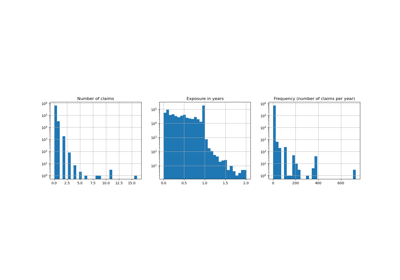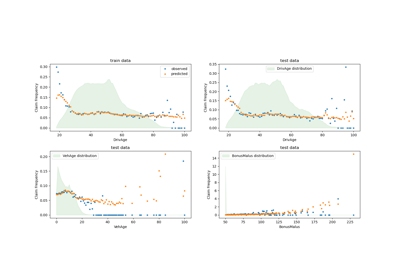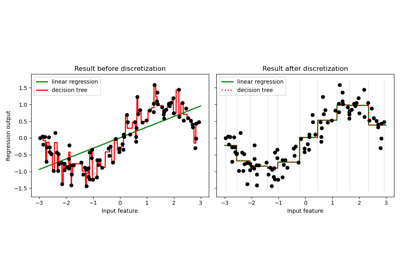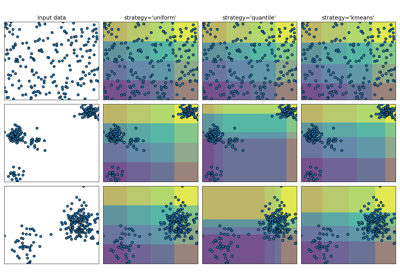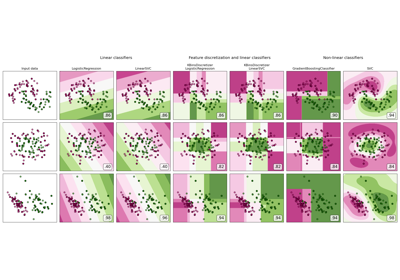# sklearn.preprocessing.KBinsDiscretizer¶

class sklearn.preprocessing.KBinsDiscretizer(n_bins=5, encode='onehot', strategy='quantile')[source]

Bin continuous data into intervals.

Read more in the User Guide.

Parameters
n_binsint or array-like, shape (n_features,) (default=5)

The number of bins to produce. Raises ValueError if n_bins < 2.

encode{‘onehot’, ‘onehot-dense’, ‘ordinal’}, (default=’onehot’)

Method used to encode the transformed result.

onehot

Encode the transformed result with one-hot encoding and return a sparse matrix. Ignored features are always stacked to the right.

onehot-dense

Encode the transformed result with one-hot encoding and return a dense array. Ignored features are always stacked to the right.

ordinal

Return the bin identifier encoded as an integer value.

strategy{‘uniform’, ‘quantile’, ‘kmeans’}, (default=’quantile’)

Strategy used to define the widths of the bins.

uniform

All bins in each feature have identical widths.

quantile

All bins in each feature have the same number of points.

kmeans

Values in each bin have the same nearest center of a 1D k-means cluster.

Attributes
n_bins_int array, shape (n_features,)

Number of bins per feature. Bins whose width are too small (i.e., <= 1e-8) are removed with a warning.

bin_edges_array of arrays, shape (n_features, )

The edges of each bin. Contain arrays of varying shapes (n_bins_, ) Ignored features will have empty arrays.

sklearn.preprocessing.Binarizer

Class used to bin values as 0 or 1 based on a parameter threshold.

Notes

In bin edges for feature i, the first and last values are used only for inverse_transform. During transform, bin edges are extended to:

np.concatenate([-np.inf, bin_edges_[i][1:-1], np.inf])


You can combine KBinsDiscretizer with sklearn.compose.ColumnTransformer if you only want to preprocess part of the features.

KBinsDiscretizer might produce constant features (e.g., when encode = 'onehot' and certain bins do not contain any data). These features can be removed with feature selection algorithms (e.g., sklearn.feature_selection.VarianceThreshold).

Examples

>>> X = [[-2, 1, -4,   -1],
...      [-1, 2, -3, -0.5],
...      [ 0, 3, -2,  0.5],
...      [ 1, 4, -1,    2]]
>>> est = KBinsDiscretizer(n_bins=3, encode='ordinal', strategy='uniform')
>>> est.fit(X)
KBinsDiscretizer(...)
>>> Xt = est.transform(X)
>>> Xt  # doctest: +SKIP
array([[ 0., 0., 0., 0.],
[ 1., 1., 1., 0.],
[ 2., 2., 2., 1.],
[ 2., 2., 2., 2.]])


Sometimes it may be useful to convert the data back into the original feature space. The inverse_transform function converts the binned data into the original feature space. Each value will be equal to the mean of the two bin edges.

>>> est.bin_edges_
array([-2., -1.,  0.,  1.])
>>> est.inverse_transform(Xt)
array([[-1.5,  1.5, -3.5, -0.5],
[-0.5,  2.5, -2.5, -0.5],
[ 0.5,  3.5, -1.5,  0.5],
[ 0.5,  3.5, -1.5,  1.5]])


Methods

 fit(self, X[, y]) Fit the estimator. fit_transform(self, X[, y]) Fit to data, then transform it. get_params(self[, deep]) Get parameters for this estimator. inverse_transform(self, Xt) Transform discretized data back to original feature space. set_params(self, \*\*params) Set the parameters of this estimator. transform(self, X) Discretize the data.
__init__(self, n_bins=5, encode='onehot', strategy='quantile')[source]

Initialize self. See help(type(self)) for accurate signature.

fit(self, X, y=None)[source]

Fit the estimator.

Parameters
Xnumeric array-like, shape (n_samples, n_features)

Data to be discretized.

yNone

Ignored. This parameter exists only for compatibility with sklearn.pipeline.Pipeline.

Returns
self
fit_transform(self, X, y=None, **fit_params)[source]

Fit to data, then transform it.

Fits transformer to X and y with optional parameters fit_params and returns a transformed version of X.

Parameters
Xndarray of shape (n_samples, n_features)

Training set.

yndarray of shape (n_samples,), default=None

Target values.

**fit_paramsdict

Returns
X_newndarray array of shape (n_samples, n_features_new)

Transformed array.

get_params(self, deep=True)[source]

Get parameters for this estimator.

Parameters
deepbool, default=True

If True, will return the parameters for this estimator and contained subobjects that are estimators.

Returns
paramsmapping of string to any

Parameter names mapped to their values.

inverse_transform(self, Xt)[source]

Transform discretized data back to original feature space.

Note that this function does not regenerate the original data due to discretization rounding.

Parameters
Xtnumeric array-like, shape (n_sample, n_features)

Transformed data in the binned space.

Returns
Xinvnumeric array-like

Data in the original feature space.

set_params(self, **params)[source]

Set the parameters of this estimator.

The method works on simple estimators as well as on nested objects (such as pipelines). The latter have parameters of the form <component>__<parameter> so that it’s possible to update each component of a nested object.

Parameters
**paramsdict

Estimator parameters.

Returns
selfobject

Estimator instance.

transform(self, X)[source]

Discretize the data.

Parameters
Xnumeric array-like, shape (n_samples, n_features)

Data to be discretized.

Returns
Xtnumeric array-like or sparse matrix

Data in the binned space.

## Examples using sklearn.preprocessing.KBinsDiscretizer¶